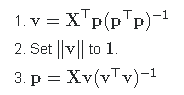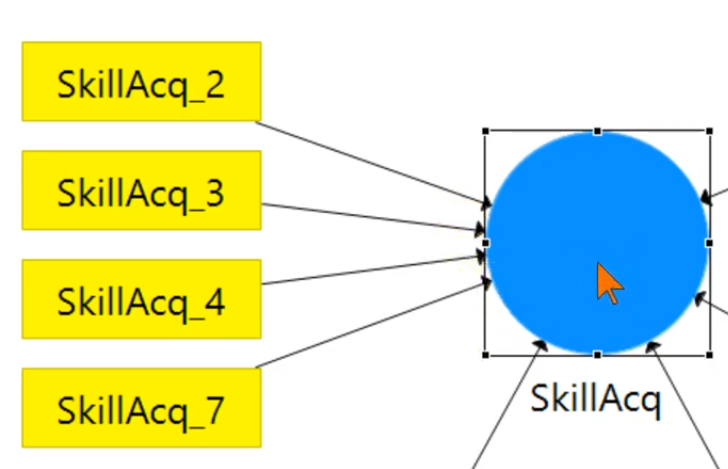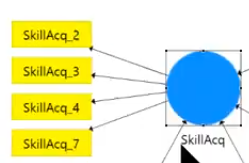# Partial Least Squares (PLS)

## Partial Least Squares Regression: (PLS)

An alternative term for PLS (and more correct according to Svante Wold) is projection to latent structures,

PLS is sometimes called “composite-based SEM”, “component-based SEM”, or “variance-based SEM”

Herman Wold, invented partial least squares (PLS) approach. He referred to this approach as NILES (Nonlinear Estimation by Iterative Least Squares Procedures) , published in 1966. focusing on the iterative nature of the procedure of estimating weights and latent variables (LVs). The “least squares” term comes from using ordinary least squares (OLS) regression to estimate other unknown parameters of a model (Wold, 1980). It seems that the term “partial” has its roots in the NILES procedures, which implemented “the idea of split the parameters of a model into subsets so they can be estimated in parts” (latent blocks of variables).

The NIPALS Algorithm (“Nonlinear Iterative vartial Least Squares”) has been developed by H. Wold at first for PCA and later-on for PLS.

Wold (1974), where the “father” of PLS presents his rationale for using the word “partial” in NIPALS definition (p. 71):

3.1.4. NIPALS estimation: Iterative OLS. If one or more variables of the model are latent, the predictor relations involve not only unknown parameters, but also unknown variables, with the result that the estimation problem becomes nonlinear. As indicated in 3.1 (iii), NIPALS solves this problem by an iterative procedure, say with steps s = 1, 2, … Each step s involves a finite number of OLS regressions, one for each predictor relation of the model. Each such regression gives proxy estimates for a sub-set of the unknown parameters and latent variables (hence the name partial least squares), and these proxy estimates are used in the next step of the procedure to calculate new proxy estimates.

PLS was introduced by Wold who used his NIPALS algorithm to implement it;

In modern expositions of PLS there is nothing “partial”: PLS looks for linear combinations among variables in X and among variables in Ythat have maximal covariance. It is an easy eigenvector problem. That’s it. See The Elements of Statistical Learning, Section 3.5.2, or e.g. Rosipal & Krämer, 2005, Overview and Recent Advances in Partial Least Squares.

NIPALS for PCA is a very simple algorithm.  Let X be a centered data matrix with observation in rows. The goal is to find the first principal axis v (eigenvector of the covariance matrix) and the first principal component p (projection of the data onto v). We initialize p randomly and then iterate the following steps until convergence:Wold viewed v and p as two [sets of] parameters, together modeling the data matrix X. The algorithm updates these parameters sequentially (steps #1 and #3), i.e. it updates only one part of the parameters at a time! Hence “partial”.

This term is remarkably misleading, because if this is “partial” then every expectation-maximization algorithm is “partial” too (in fact, NIPALS can be seen as a primitive form of EM, see Roweis 1998).

Ref: Garson, G. D. (2016). Partial Least Squares: Regression and Structural Equation Models. Asheboro, NC: Statistical Associates Publishers.

#————————————

### 2013 Against PLS

Rönkkö, Mikko, and Joerg Evermann. 2013. “A Critical Examination of Common Beliefs About Partial Least Squares Path Modeling.” Organizational Research Methods 16 (3): 425–48. https://doi.org/10.1177/1094428112474693.

### 2014 for PLS

Henseler, Jörg, Theo K. Dijkstra, Marko Sarstedt, Christian M. Ringle, Adamantios Diamantopoulos, Detmar W. Straub, David J. Ketchen, Joseph F. Hair, G. Tomas M. Hult, and Roger J. Calantone. 2014. “Common Beliefs and Reality About PLS: Comments on Rönkkö and Evermann (2013).” Organizational Research Methods 17 (2): 182–209. https://doi.org/10.1177/1094428114526928.

Lowry, P. B., and J. Gaskin. 2014. “Partial Least Squares (PLS) Structural Equation Modeling (SEM) for Building and Testing Behavioral Causal Theory: When to Choose It and How to Use It.” IEEE Transactions on Professional Communication 57 (2): 123–46. https://doi.org/10.1109/TPC.2014.2312452.

https://ieeexplore.ieee.org/document/6803892

PLS regression is particularly suited when the matrix of predictors has more variables than observations, and when there is multicollinearity among X values. By contrast, standard regression will fail in these cases (unless it is regularized). Wikipedia

### 2016 Against PLS

Rönkkö, Mikko, Cameron N. McIntosh, John Antonakis, and Jeffrey R. Edwards. 2016. “Partial Least Squares Path Modeling: Time for Some Serious Second Thoughts.” Journal of Operations Management 47–48 (1): 9–27. https://doi.org/10.1016/j.jom.2016.05.002.

### 2017 for PLS

Akter, Shahriar, Samuel Fosso Wamba, and Saifullah Dewan. 2017. “Why PLS-SEM Is Suitable for Complex Modelling? An Empirical Illustration in Big Data Analytics Quality.” Production Planning & Control 28 (11–12): 1011–21. https://doi.org/10.1080/09537287.2016.1267411.

Rigdon, Edward E., Marko Sarstedt, and Christian M. Ringle. 2017. “On Comparing Results from CB-SEM and PLS-SEM: Five Perspectives and Five Recommendations.” Marketing ZFP 39 (3): 4–16. https://doi.org/10.15358/0344-1369-2017-3-4.

Hair, Joseph F., G. Tomas M. Hult, Christian M. Ringle, Marko Sarstedt, and Kai Oliver Thiele. 2017. “Mirror, Mirror on the Wall: A Comparative Evaluation of Composite-Based Structural Equation Modeling Methods.” Journal of the Academy of Marketing Science 45 (5): 616–32. https://doi.org/10.1007/s11747-017-0517-x.

Rönkkö, Mikko Writes PLS package for R
https://cran.r-project.org/web/packages/matrixpls/index.html

### 2018 for PLS

Theo K. Dijkstra PhD thesis (1981, NWO grant, supervisor H. Wold) analyzed PLS, GLS and LISREL. Fulbright scholar at UCLA (Dept. of Psychology, P. M. Bentler, 1982). Taught Econometrics, Statistics, Portfolio Theory, and Mathematical Finance at the University of Groningen (RuG).

This article introduces a new consistent variance-based estimator called ordinal consistent partial least squares (OrdPLSc). OrdPLSc completes the family of variance-based estimators consisting of PLS, PLSc, and OrdPLS and permits to estimate structural equation models of composites and common factors if some or all indicators are measured on an ordinal categorical scale. A Monte Carlo simulation (N =500) with different population models shows that OrdPLSc provides almost unbiased estimates. If all constructs are modeled as common factors, OrdPLSc yields estimates close to those of its covariance-based counterpart, WLSMV, but is less efficient. If some constructs are modeled as composites, OrdPLSc is virtually without competition.

Schuberth, Florian, Jörg Henseler, and Theo K. Dijkstra. 2018. “Partial Least Squares Path Modeling Using Ordinal Categorical Indicators.” Quality & Quantity 52 (1): 9–35. https://doi.org/10.1007/s11135-016-0401-7.

Aguirre-Urreta, M. I., & Rönkkö, M. (2018). Statistical Inference with PLSc Using Bootstrap Confidence Intervals. MIS Quarterly, 42 (3), 1001-1020. doi:10.25300/MISQ/2018/13587

Happy Ending 🙂

#——————

“If you want to see how well a combination of a set of variables
can predict a response variable, use plsr. plsr will produce a better prediction
of the response variable, because that is what it is designed to do, but it will often
be more difficult to interpret.” (Wright, Daniel B., and Kamala London, 2009. , 82)

to model a response variable when there are a large number of predictor variables, and those predictors are highly correlated or even collinear.

PLSr does take the response variable into account, and therefore often leads to models that are able to fit the response variable with fewer components.

http://www.mathworks.com/help/stats/examples/partial-least-squares-regression-and-principal-components-regression.html?requestedDomain=www.mathworks.com

Partial least square (PLS) is a supervised alternative to PCA. PLS assigns higher weight to variables which are strongly related to response variable to determine principal components.

Traditional SEM will not be of value when as we don’t have a good feel or theory to make assumptions (or when we don’t want to make assumptions) on the latent structure.

http://gastonsanchez.com/

https://www.r-bloggers.com/partial-least-squares-regression-in-r/

https://www.analyticsvidhya.com/blog/2016/03/practical-guide-principal-component-analysis-python/

#### 2. Ordinal PLS

Provides (weighted) Partial least squares Regression for generalized linear models and repeated k-fold cross-validation of such models using various criteria. It allows for missing data in the ex-planatory variables. Bootstrap confidence intervals constructions are also available.

https://cran.r-project.org/web/packages/plsRglm/plsRglm.pdf

https://cran.r-project.org/web/packages/plsRglm/plsRglm.pdf

https://cran.r-project.org/web/packages/plsRglm/index.html

#### 3. SEM PLS

https://cran.r-project.org/web/packages/semPLS/vignettes/semPLS-intro.pdf

Partial Least Squares (PLS) (also known as Projection to Latent Structure)

It many ways, PLS can be regarded as a substitute for the method of multiple regression, especially when the number of predictor variables is large. In such cases, there is seldom enough data to construct a reliable model that can be used for predicting the dependent data Y from the predictor variables X. Instead, we get a model that can perfectly fit the training data while performing poorly on unseen samples. This problem is known as over-fitting (Bishop 1995).

PLS alleviates this problem by adopting the “often correct” assumption that, although there might be a large number of predictor variables, the data may actually be much simpler and can be modeled with the aid of just a handful of components (also known as latent). This in fact is the same technique used by PCA for representing the X variables with the aid of a lesser number of principal components.

The idea is to construct a set of components that accounts for as much as possible variation in the data while also modeling the Y variables well.

The technique works by extracting a set of components that transforms the original data X to a set of t-scores T (as in PCA). Similarly, Y is used to define another set of components known the u-scores U. The t-scores are then used to predict the u-scores, which in turn are used to predict the response variables Y. This multistage process is hidden, and all we see as the outcome is that for a set of predictor variables X, PLS predicts a set of relating responses Y. Thus, PLS works just as any other regression model.http://documentation.statsoft.com/STATISTICAHelp.aspx?path=MSPC/PCAandPLSTechnicalDetails

http://stats.stackexchange.com/questions/84185/lasso-to-identify-important-variables-in-ordered-logistic-regression

#### 4) Consistant PLS

A formative construct or composite refers to an index of a weighted sum of variables.

In a formative construct, the indicators cause the construct,In a more conventional latent variables, sometimes called reflective constructs, the indicators are caused by the latent variable. For reflective constructs Consistent PLS should be usedConsistant PLS can be used for both formative and reflective constructs.

### PLS SOFTWARE

http://www.plsmodeling.com/resources/software.html

https://www.smartpls.com/smartpls2

#### in R:

https://cran.r-project.org/web/packages/pls/index.html

See also Gaston Sanchez’s (package developer) perfect manual on PLS in general and plspm in particular: http://gastonsanchez.com/PLS_Path_Modeling_with_R.pdf.

https://cran.r-project.org/web/packages/semPLS/index.html

https://cran.r-project.org/web/packages/seminr/vignettes/SEMinR.html

#——-

XLSTAT
WarpPLS
PLS-GUI
Minitab
PLS-Graph
VisualPLS